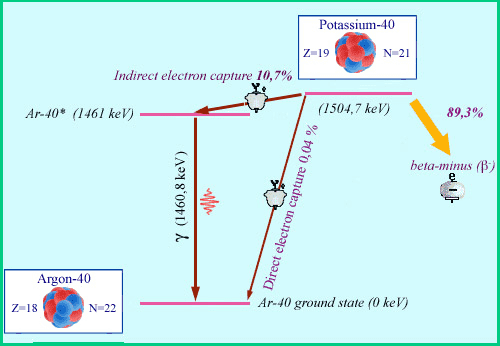# Does K prefer K capture?

• I
snorkack
TL;DR Summary
Handling of spin in electron capture
K-40 often captures electrons. This is why Earth atmosphere is full of Ar-40.
Where does K-40 capture electrons from?
The problem is that K-40 has spin 4. Ar-40 has spin 0.
How are the 4 spin units handled?
The spins of the electron and neutrino combine to at most 1. This leaves 3 spin units to handle. How?
By emitting the neutrino with an orbital angular momentum? Or by capturing electron with orbital angular momentum?
Orbital angular momentum is not found in K-shell (it is 1s orbital, no angular momentum). Does K-40 preferentially capture outer electrons which do have angular momentum?

•ohwilleke and vanhees71

Homework Helper
Gold Member
2022 Award
Summary: Handling of spin in electron capture

K-40 often captures electrons. This is why Earth atmosphere is full of Ar-40.
About 11% of K-40 decays are due to electron capture.
I would not say that the atmosphere is full of Ar-40, that is a bit of an exaggeration.

Where does K-40 capture electrons from?
From the electons bound to the nuclei, they have a small probability to be located close enough to the nucleus such that the process ##e^- + p \to n + \nu + \gamma## occurs.
Neutron remain in nucleus, Ar-40 is formed.
The photon is not emitted directly, the Ar-40 is formed in an excited state with some non-zero angular momentum. The emission of the photon will carry away that excess angular momentum so that the final state nuclei has spin 0. It is also thanks to this photon that we can use K-40 for radioactive dating (give it a try next valentines day!)
Does K-40 preferentially capture outer electrons which do have angular momentum?
No, because such electron states have extremely low probability to be near the nuclei.•vanhees71
Gold Member
we can use K-40 for radioactive dating (give it a try next valentines day!)
Har! Har! Har!Har! Har! Har!